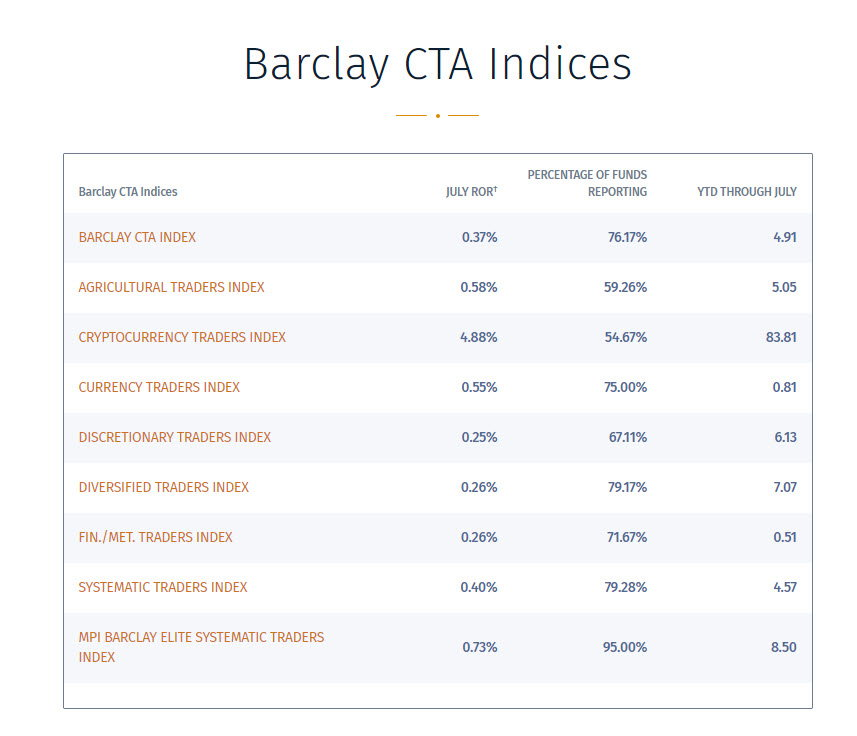A Question of Balance

We released the Ranger Alpha strategies at the beginning of September 2019. It is important for any prospective client to know that the performance that we track is completely OUT-OF-SAMPLE since that time.

For those of you who don’t know of me and my trading achievements, these numbers might seem a bit unbelievable. I understand. This might even be true for those of you who do.

That is one reason why we wanted to explain them and their leverage a bit.

But before I do that, I want to assure you that these exceptional returns are real. If you are a bit skeptical, take a look at our Testimonials page or watch our one of our more recent videos with Mike and Trevor.

In any case let’s talk about leverage. We have not changed this since their release so as to preserve continuity of the record.

The way that one leverages a portfolio of uncorrelated strategies is also a bit different. Ranger Alpha is a portfolio of six such strategies on the eMini. One of the major benefits of such a portfolio of strategies on one market is that of minimizing drawdown. Just the same as it would be for a portfolio of different markets.

As a result of this strategy diversification, it is not that often that all of these strategies are in positions in the same direction at the same time. As a matter of fact, we have not seen it happen once yet.

So, whereas the maximum margin could be about \$66,000, it has never yet happened.

Given that, this maximum margin would be constitute a little over 50% leverage on a \$125,000 account, However, we have seen as much as three, occasionally four, such positions which then puts the margin at around 25% leverage.

That might still be a bit much for some people, so we would then recommend either reducing the number of strategies or increasing the account size.

Let’s see what happens if we do that. Let’s double the account size to \$250,000. This would reduce maximum possible leverage to 25% and typical to 12%.

The reported cumulative return of Ranger Alpha would then be 151%. I can live with that.

Now, let’s take a look at the type of leverage that a commodity trading advisor would typically be expected to use.

The leverage of a CTA is measured by what is called the margin-to-equity ratio (MER). Typically, this is expected to be in a range from 15 to 25%. Let’s take the midpoint or 20% MER. This is not a requirement but rather a best practice and an industry standard.

For those of you not familiar with MER, let me explain. It simply means that the total margin committed at any time should not exceed 20% of the account size traded. So, in a \$125,000 account that means that margin should not exceed \$25,000.

What would it look like if we traded Ranger Alpha at this leverage?

At a position maximum of six, that would be margin of \$66,000. It would call for an account, rounding up, of \$330,000. Since the typical margin rarely exceeds \$33,000 this gives us a large margin of error. Let’s go with the conservative approach.

The cumulative return of Ranger Alpha as of 8-16-2021 was \$377,615. That is a return of 114% on a \$3330,000 account. That also is not too shabby.

Finally, let’s take a look at the annualized rate of return for each of these leverage levels.

Ranger Alpha was released 9/2019. That is bit more than 23 months.

So for the –

• \$125,000 account that is an ARR of 158%
• \$250,000 account that is an ARR of 79%
• \$300,000 account that is an ARR of 59%.

As a basis for comparison, the Barclay CTA Index for all types of CTA year to date through July is +4.91 %. Of course, that is an index of all CTA’s but it is a widely accepted basis of comparison. You can see the table below.